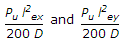# Civil Engineering - UPSC Civil Service Exam Questions

11.

The given figure shows the arrow diagram for a particular project. The arrow 'A' is known as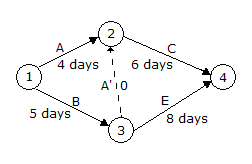A. critical activity B. sub-critical activity C. logic arrow D. dummy activity

Explanation:

No answer description available for this question. Let us discuss.

12.

In a plane strain problem in XY plane, the shear strain = 12 x 10-6, and the normal strain in X and Y direction = 0, For this state of strain, what is the diameter of the Mohr's Circle of strain ?

 A. 6 x 10-6 B. 8 x 10-6 C. 12 x l0-6 D. 24 x l0-6

Explanation:

No answer description available for this question. Let us discuss.

13.

A horizontal fixed beam is fixed at both it ends A and B. During loading, the right support sinks by an amount δ. Flexural rigidity of the beam is uniform and is equal to EI. Length of the beam is L. What is the moment developed at the centre of the beam due to sinking of the support ?

 A. 6 EI δ/L2 B. zero C. 3 EI δ/L2 D. 12 EI δ/L2

Explanation:

No answer description available for this question. Let us discuss.

14.

A lighthouse is visible just above the horizon at a certain station at the sea level. The distance between the station and the lighthouse is 40 km. The height of the lighthouse is approximately

 A. 187 m B. 137.7 m C. 107.7 m D. 87.3 m

Explanation:

No answer description available for this question. Let us discuss.

15.

Which of the following are the additional moments considered for design of slender compression member in lieu of deflectiion x and y directions ?(Where Pu is axial load; lex and ley are effective lengths in respective directions; D depth of section ⊥ to major axis; b width of the member)

 A.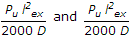B.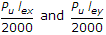C.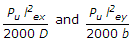D.